# Better than Unit Tests? Idris Proofs

`namespace Numbers  data Nat : Type where    Zero : Numbers.Nat    Successor : Numbers.Nat -> Numbers.Nat  decr : Numbers.Nat -> Numbers.Nat  decr Zero = Zero  decr (Successor x) = x  incr : Numbers.Nat -> Numbers.Nat  incr Zero = Successor Zero  incr (Successor x) = Successor (Successor x)`
`One : Numbers.NatOne = Successor ZeroTwo : Numbers.NatTwo = Successor OneThree : Numbers.NatThree = Successor Two`
`proofIncrementOne : incr One = TwoproofIncrementOne = ReflproofDecrementOne : decr One = ZeroproofDecrementOne = ReflproofDecrementZero : decr Zero = ZeroproofDecrementZero = Refl`
`plus : Numbers.Nat -> Numbers.Nat -> Numbers.Natplus Zero b = bplus (Successor a) b = Successor(plus a b)syntax [x] "+" [y] = Numbers.plus x y`
`proofTwoPlusOne : (Two + One) = ThreeproofTwoPlusOne = Refl`
`proofZeroPlusN : (n : Numbers.Nat) -> (Zero + n) = nproofZeroPlusN n = Refl`
• prove a base case (in this instance `n = Zero`) some property (`p`)holds true. In this instance the property is `n + 0 = n`.
• prove for some successor to the base case (in this instance `n = Successor k`) the property (`p`) also holds true; said another way if `p(n)` holds true then `p(Successor n)` also holds true.
• conclude the property holds true for all
`proofNPlusZero : (n : Numbers.Nat) -> (n + Zero) = nproofNPlusZero Zero = ReflproofNPlusZero (Successor k) =    let inductiveNPlusZero = proofNPlusZero k in      rewrite inductiveNPlusZero in Refl`
`lemmaPlusCommutesZeroM : (m: Numbers.Nat) -> m = plus m Zero  lemmaPlusCommutesZeroM (Successor k) =    let inductiveCummativeM = lemmaPlusCommutesZeroM k in      rewrite inductiveCummativeM in RefllemmaPlusCommutesZeroKM : (k, m: Numbers.Nat) ->                          Successor (plus m k) = plus m (Successor k)  lemmaPlusCommutesZeroKM k Zero = Refl  lemmaPlusCommutesZeroKM k (Successor j) =    rewrite lemmaPlusCommutesZeroKM k j in ReflproofPlusCommutative : (n, m : Numbers.Nat) -> (n + m) = (m + n)  proofPlusCommutative Zero Zero = Refl  proofPlusCommutative Zero m = lemmaPlusCommutesZeroM m  proofPlusCommutative (Successor n) m =    rewrite proofPlusCommutative n m in lemmaPlusCommutesZeroKM n m`

--

--

--

## More from Todd Brown

A 25 year software industry veteran with a passion for functional programming, architecture, mentoring / team development, xp/agile and doing the right thing.

Love podcasts or audiobooks? Learn on the go with our new app.

## Recommended from Medium## DevOps: The Infinite Mindset## Daily Stand-Up as you like it## Java Date Formatting and Utilization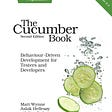## Evaluation: Run It!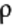## How booting work in operating system?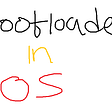## Array Order and Permute() in MATLAB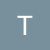## Todd Brown

A 25 year software industry veteran with a passion for functional programming, architecture, mentoring / team development, xp/agile and doing the right thing.

## My vision about outside-in testing as a Backend Developer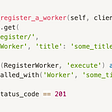## DB-Lock-Issues with Transactional REQUIRES_NEW — More Spring/Java transaction handling pitfalls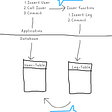## How bad models ruin an API## 4 Things I’ve learned as a Software Engineer at ServiceRocket